Next: Sample Codes for Using Up: How FFTEASY works Previous: FFTR1: One-Dimensional Real Fourier

## FFTRN: Multi-Dimensional Real Fourier Transforms

The structure of fftrn is almost identical to fftr1 and the basic equations used are the same. The key difference comes from the fact that in more than one dimension the elementcan no longer simply be found at the location. Rather the index must be reversed fromtoseparately for each dimension. Likewise in the one-dimensional caseonly needed to include non-negative value ofwhereas in this case that is true only in the last dimension.

This indexing is particularly tricky since fftrn treats the data as a one-dimensional array. (There is no practical way to treat the data as a multi-dimensional array without knowing in advance how many dimensions are going to be used.) Fortunately there is a simple solution to this problem. The function fftrn defines an array calledthat keeps track of the separate indices in all the dimensions. At the bottom of the main loop these indices are incremented, advancing the last one until it reaches its maximum and then starting it over and incrementing the next to last one, and so on. At the top of the main loop is a call to the function find_index, which finds the one-dimensional array index that corresponds to these different multi-dimensional indices. More importantly, the function find_index also finds the one-dimensional index corresponding to the negative of the frequencies indicated by. From there the implementation is almost identical to fftr1. The inner loop runs over the last dimension and applies the exact same formulas as fftr1 to calculate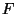, the desired transform, from, the output of fftcn. I leave it as an exercise for the reader to show that these formulas are still valid provided the frequencies in all but the last dimension are replaced by their corresponding negative frequencies as shown.

Theand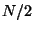(Nyquist) frequencies present a problem. In one dimension these both had to be real because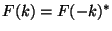. In multiple dimensions, however, it is no longer true that all values offor which the last index areorare real. To illustrate what I mean let me use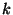to indicate the frequency in the last dimension and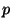to indicate the frequency components in all other dimensions. Now the symmetry is simply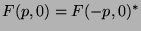. This means that applying equation  to the case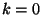will no longer yield a real number as it did in the one-dimensional case and socan no longer be packaged withinto one complex array index. So fftrn takes as input an arraythat it uses for the valuesand uses both the real and imaginary parts of the first elements of the arrayfor. In practice this means that the output is redundant because it will contain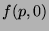and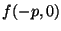, which are complex conjugates of each other (and likewise forandin).

As with fftr1 the algebra for the inverse transform turns out to be virtually identical to the forward case, and once again I leave the details to the reader to verify. Note that it is up to the user to make sure that the symmetries noted above are obeyed by the input to the inverse transform. In section [4.3] I provide sample code for generating data with all the correct symmetries in three dimensions. You can simply cut and paste this sample code into your own program and substitute whatever function you want to generate in frequency space.Next: Sample Codes for Using Up: How FFTEASY works Previous: FFTR1: One-Dimensional Real Fourier